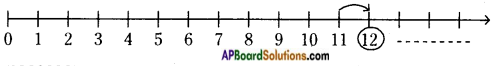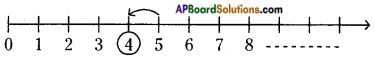# AP Board 6th Class Maths Solutions Chapter 2 Whole Numbers Unit Exercise

AP State Syllabus AP Board 6th Class Maths Solutions Chapter 2 Whole Numbers Unit Exercise Textbook Questions and Answers.

## AP State Syllabus 6th Class Maths Solutions 2nd Lesson Whole Numbers Unit Exercise

Question 1.
Choose the appropriate symbol from < or > and place it in the blanks.
i) 8 ……. 7
ii) 5 ……. 2
iii) 0 ……. 1
iv) 10 ……. 5
i) 8 …. > …. 7
ii) 5 …. > …. 2
iii) 0 …. < …. 1
iv) 10 …. > …. 5Question 2.
Present the successor of 11 and predecessor of 5 on the number line.
i)Successor of 11 is 12.

ii)Predecessor of 5 is 4.

Question 3.
Which of the statements are true ( T ) and which are false ( F ). Correct the false statements.
i) There is a natural number that has no predecessor. ( T )
ii) Zero is the smallest whole number. ( T )
iii) A whole number on the left of another number on the number line, is greater than that number. (F)
i) There is a natural number that has no predecessor. ( T )
ii) Zero is the smallest whole number. ( T )
iii) A whole number on the left of another number on the number line, is greater than that number. ( F )Question 4.
Give the results without actually performing the operations, using the given information.
i) 28 × 19 = 532 , then 19 × 28 =
ii) a × b = c , then b × a =
iii) 85 + 0 = 85 , then 0 + 85 =
i) 28 × 19 = 532 , then 19 × 28 = 532
ii) a × b = c , then b × a = c
iii) 85 + 0 = 85 , then 0 + 85 = 85

Question 5.
Find the value of the following:
i) 368 × 12 + 18 × 368
ii) 79 × 4319 + 4319 × 11
i) 368 × 12 + 18 × 368 – 368 × 12 + 368 × 18
Distributive property of multiplication over addition.
= 368 × (12 + 18)
= 368 × 30 = 11040

ii) 79 × 4319 + 4319 × 11
= 79 × 4319 + 11 × 4319
Distributive property of multiplication over addition.
= (79 + 11) × 4319
= 90 × 4319;
= 388710

Question 6.
Chandana and Venu purchased 12 note books and 10 note books respectively. The cost of each note book is Rs. 15. Then how much amount should they pay to the shopkeeper?
Number of note books purchased by Chandana = 12
Number of note books purchased by Venu = 10
Total number of note books purchased together = 12 + 10
Cost of each note book = Rs. 15
Cost of (12 + 10) note books = (12 + 10) × 15
= 22 × 15
The amount paid to the shopkeeper = Rs. 330Question 7.
Match the following.
i) 3 + 1991 + 7 = 3 + 7 + 1991                        [ ]                A) Additive identity
ii) 2 × 68 × 50 = 2 × 50 × 68                           [ ]                B) Multiplicative identity
iii) 1                                                                  [ ]                C) Commutative under addition
iv) 0                                                                  [ ]                D) Distributive property of multiplication over addition
v) 879 × (100 + 30) = 879 × 100 + 879 × 30  [ ]                E) Commutative under multiplication
i) C
ii) E
iii) B
iv) A
v) D

Question 8.
Study the pattern:
91 × 11 × 1 = 1001
91 × 11 × 2 = 2002
91 × 11 × 3 = 3003
Write next seven steps. Check, whether the result is correct.